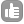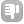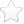21 When positive and negative coupling constants occur in NMR? and why? What are the spin states of the nuclei and the bond electrons for each situation? An alpha proton coupled to other proton decrease or increase its frequency of resonance for a 1J, 2J and 3J? Thank you so much asked Jun 04 '10 at 15:25Ligia 21Evgeny Fadeev 577●12The Hamiltonian describing the interaction between the spin operators of two nuclei can be written in the following form: IA . J . IB IA and IB are the spin operators of the two nuclei that are interacting and J is a tensor. In solution, due to rapid averaging, the contribution to the Hamiltonian from this interaction can be written as: J IA . IB In solution NMR spectroscopy, J is a scalar and can be either positive, negative or zero. If J is positive then, you have a positive coupling constant and if J is negative then you have a negative coupling constant. Furthermore, if the coupling is weak (i.e., the chemical shift separation between the resonance frequencies of A and B is much greater than the magnitude of the coupling constant J), the contribution to the Hamiltonian from the J-coupling can be written as: J IAz . IBz The physical mechanism of interaction between two spins, mediated through electrons (the J-coupling interaction) can be explained by valence bond theory. Essentially, the magnitude of the coupling constant depends upon the probability of electrons at the nucleus and the sign depends on the nature of the two nuclei that are coupled and the manner in which the interaction is mediated by the intervening electrons. The following qualitative explanation is sometimes used to explain the common observation that for two nuclei having the same sign of magnetogyric ratio, 1J coupling is positive, 2J coupling is negative and 3J coupling is positive. Let A+ indicate that spin A is in a state where mz is positive and A- indicate a state with negative value of mz. Assume that in the stable configuration two electrons in the same molecular orbital will have different signs of mz (E+.E- or E-.E+) due to Pauli exclusion principle and that for two electrons interacting in different bonds the lowest energy spin configuration is one where the interaction electrons have the same sign of mz (E+..E+ or E-..E-). Assume that the magnetogyric ratios of nuclei A and B are both positive e.g., 1H or 13C. With these assumptions we can write down the favorable energy configurations for one bond, two bond and three bond couplings: The most stable configuration for two nuclear spins separated by one bond is: A+ .. E-.E+ .. B-. i.e., mzA.mzB is negative (A and B are antiparallel). If, mzA.mzB is positive you would expected a higher energy configuration. (Positive J-coupling) The most stable configuration for two nuclear spins separated by two bonds is: A+ .. E-.E+.. E+.E- .. B+ Therefore, the lowest energy configuration corresponds to the case where mzA.mzB is positive and the other orientation i.e., mzA.mzB negative is of higher energy. (Negative Jcoupling) Similarly, for the most stable configuration for two nuclear spins separated by three bonds is: A+ .. E-.E+.. E+.E- ..E-E+ .. B- Therefore, the lowest energy configuration corresponds to the case where mzA.mzB is negative and the other orientation (mzA.mzB positive) is of higher energy. (Positive J coupling) The above explanation is only qualitative and CERTAINLY DOES NOT explain all aspects of the sign of J-coupling. It is only included to give an intuitive feel for explaining the factors that can change the sign of the coupling constant. The references included at the bottom should be consulted for a rigorous description of this phenomena. Regarding the second question. The resonance frequency is NOT changed by J-coupling (of alpha or of any other nuclear spin, whether 1J, 2J, ..). J-coupling is usually observable in the 1D-spectrum as a splitting of the resonance signal into a multiplet. Some relevant References: Electron Coupled Interactions between Nuclear Spins in Molecules. Norman F. Ramsey. Phys. Rev. 91, 303–307 (1953). Valence Bond Interpretation of Electron Coupled Nuclear Spin Interactions. Martin Karplus and D. H. Anderson. J. Chem. Phys. 30, 6 (1959) Contact Electron Spin Coupling of Nuclear Magnetic Moments. Martin Karplus. J. Chem. Phys. 30, 11 (1959)). I believe the book by James Keeler would be a good introduction to the basic theory of NMR Hamiltonians, Spin Operators, etc. (Understanding NMR Spectroscopy. James Keeler. Edition 2. John Wiley & Sons, 2010) answered Jun 13 '10 at 03:42